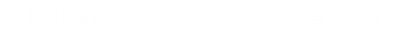Working with Formulas

The Formula form field property lets you define the content of a field based on specific logical operations performed on one or more other text fields. Text or Area Text fields can be used as operands in formulas. Each field is defined as either a String operand or a Number operand, as follows:

• Fields with a validation type of 'Numeric' or 'Numeric decimal' are defined as Number operands.
• Fields with validation types other than 'Numeric' or 'Numeric decimal' are defined as String operands.

Supported Operations for Number Operands

Operation

Operator

Example

Concatenation +
Conversion convert
Case Statement case